# Patterns and Sequences Patterns and Sequences Patterns refer

• Slides: 20Patterns and SequencesPatterns and Sequences Patterns refer to usual types of procedures or rules that can be followed. Patterns are useful to predict what came before or what might come after a set a numbers that are arranged in a particular order. This arrangement of numbers is called a sequence. For example: 3, 6, 9, 12 and 15 are numbers that form a pattern called a sequence The numbers that are in the sequence are called terms.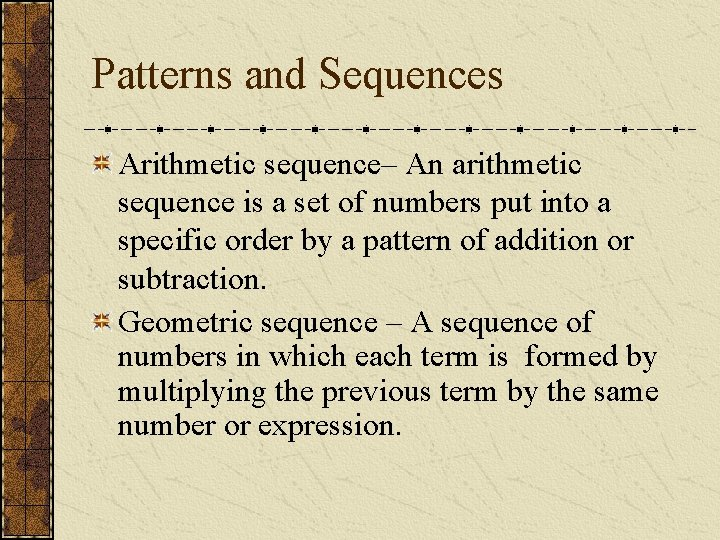Patterns and Sequences Arithmetic sequence– An arithmetic sequence is a set of numbers put into a specific order by a pattern of addition or subtraction. Geometric sequence – A sequence of numbers in which each term is formed by multiplying the previous term by the same number or expression.Arithmetic Sequence Find the next three numbers or terms in each pattern. Look for a pattern: usually a procedure or rule that uses the same number or expression each time to find the next term. The pattern is to add 5 to each term. The next three terms are:Arithmetic Sequence Find the next three numbers or terms in each pattern. Look for a pattern: usually a procedure or rule that uses the same number or expression each time to find the next term. The pattern is to add the integer (-3) to each term. The next three terms are: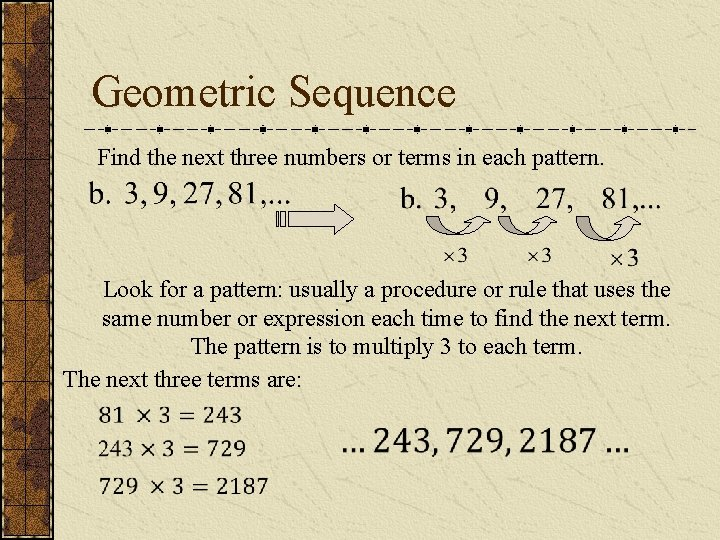Geometric Sequence Find the next three numbers or terms in each pattern. Look for a pattern: usually a procedure or rule that uses the same number or expression each time to find the next term. The pattern is to multiply 3 to each term. The next three terms are: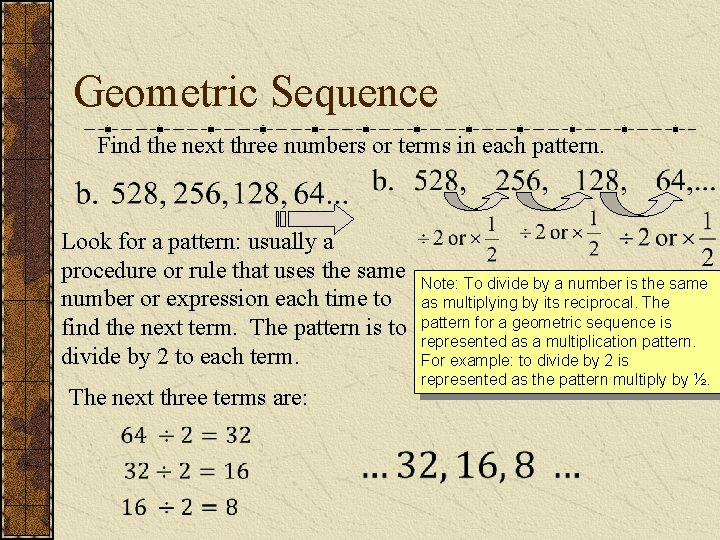Geometric Sequence Find the next three numbers or terms in each pattern. Look for a pattern: usually a procedure or rule that uses the same number or expression each time to find the next term. The pattern is to divide by 2 to each term. The next three terms are: Note: To divide by a number is the same as multiplying by its reciprocal. The pattern for a geometric sequence is represented as a multiplication pattern. For example: to divide by 2 is represented as the pattern multiply by ½.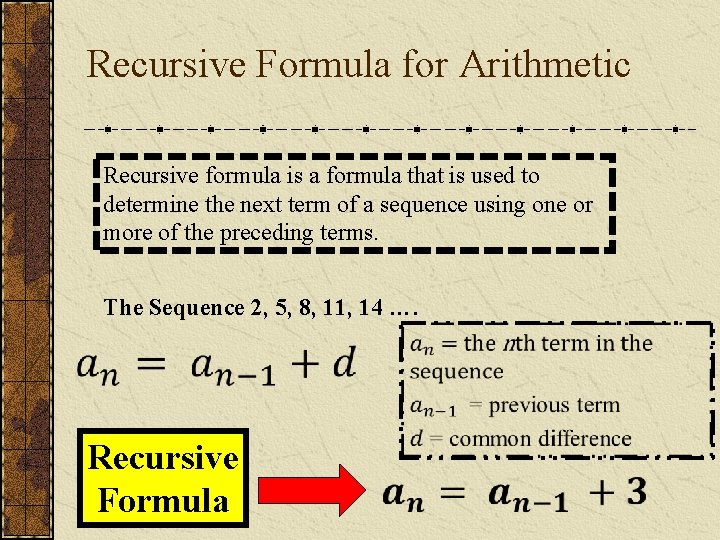Recursive Formula for Arithmetic Recursive formula is a formula that is used to determine the next term of a sequence using one or more of the preceding terms. The Sequence 2, 5, 8, 11, 14 …. Recursive Formula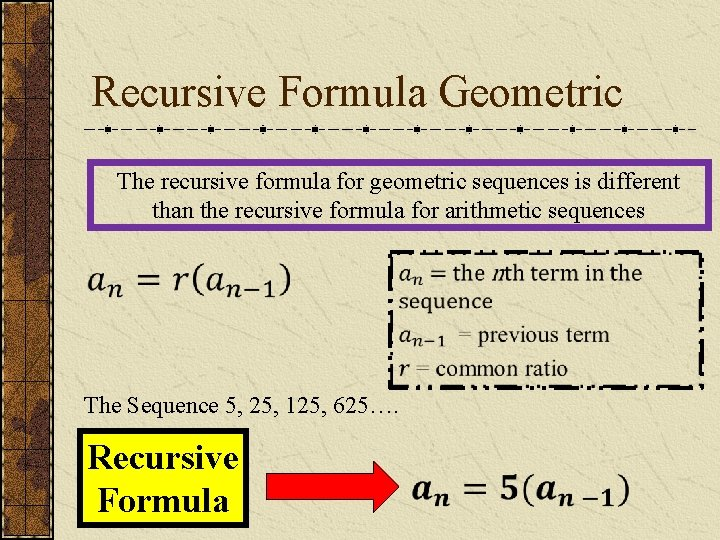Recursive Formula Geometric The recursive formula for geometric sequences is different than the recursive formula for arithmetic sequences The Sequence 5, 25, 125, 625…. Recursive FormulaWrite the Recursive Formula for Arithmetic sequencesWrite the Recursive Formula for Geometric sequences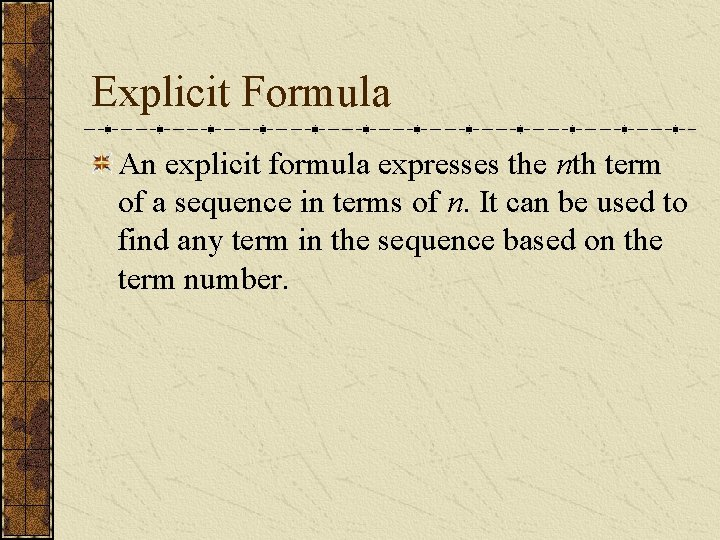Explicit Formula An explicit formula expresses the nth term of a sequence in terms of n. It can be used to find any term in the sequence based on the term number.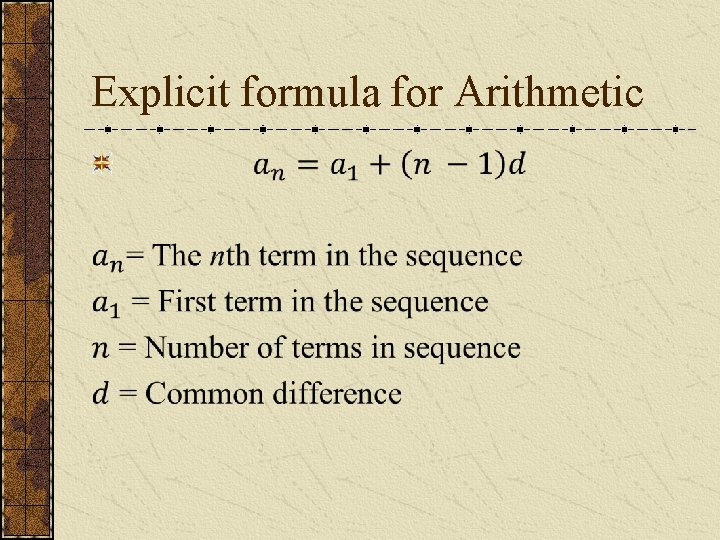Explicit formula for Arithmetic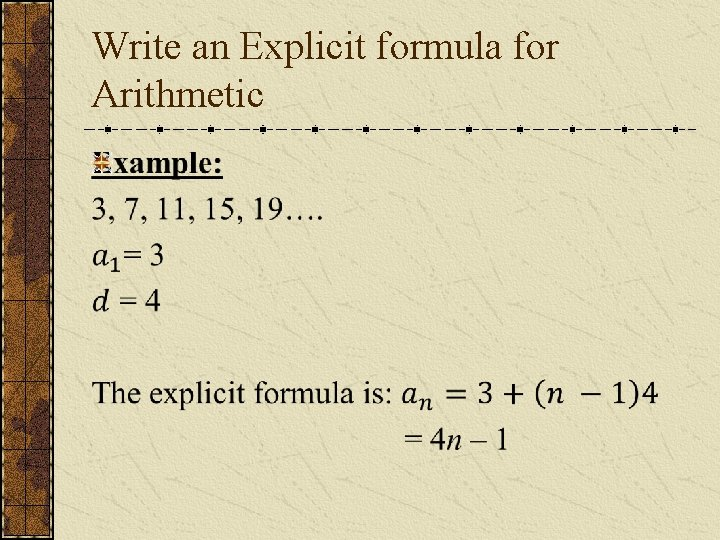Write an Explicit formula for Arithmetic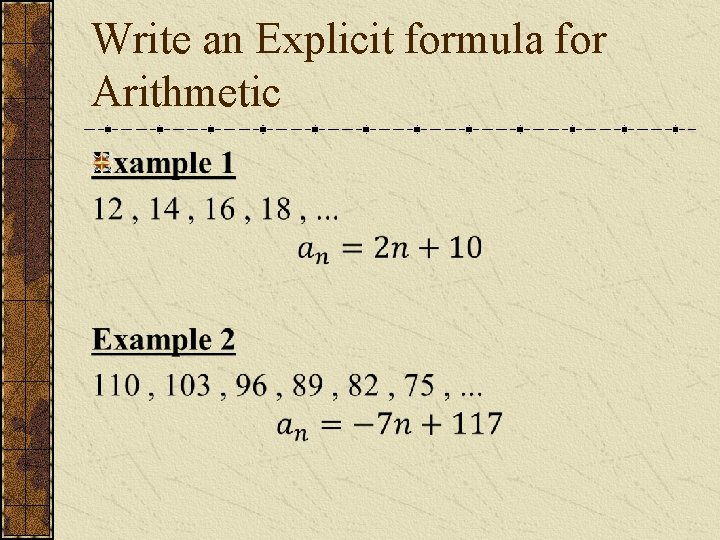Write an Explicit formula for ArithmeticFinding the nth term in the Explicit formula for Arithmetic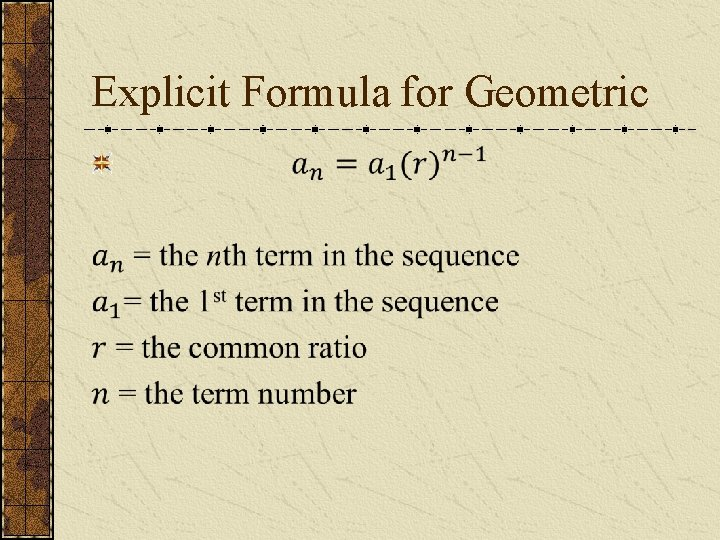Explicit Formula for Geometric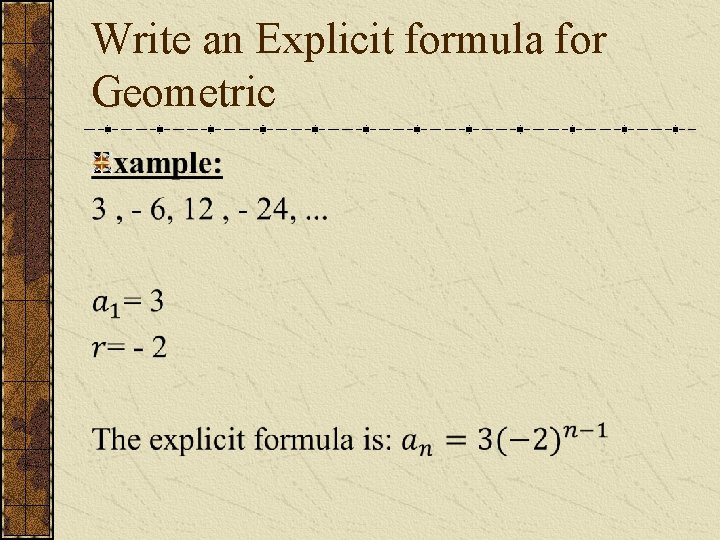Write an Explicit formula for GeometricWrite the Explicit formula for Geometric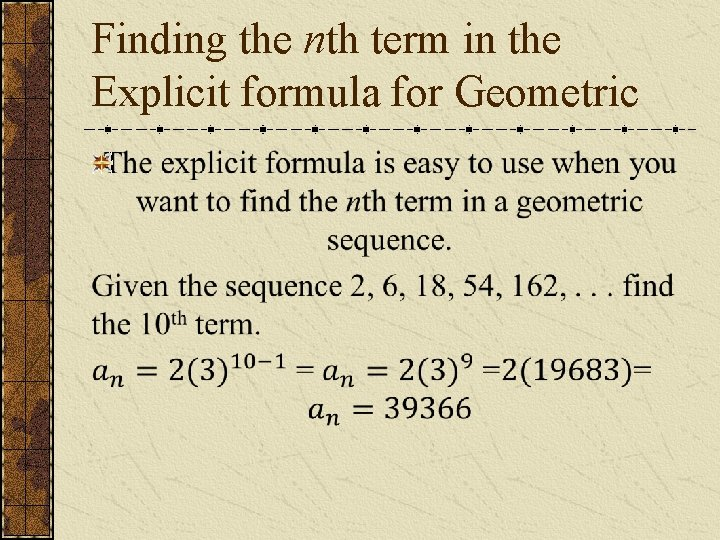Finding the nth term in the Explicit formula for Geometric